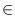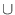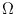Next: Maximum Flow ( max_flow Up: Graph Algorithms Previous: Basic Graph Algorithms (   Contents   Index

# Shortest Path Algorithms ( shortest_path )

Let G be a graph, s a node in G, and c a cost function on the edges of G. Edge costs may be positive or negative. For a node v let(v) be the length of a shortest path from s to v (more precisely, the infimum of the lengths of all paths from s to v). If v is not reachable from s then(v) = +and if v is reachable from s through a cycle of negative cost then(v) = -. Let V+, Vf, and V- be the set of nodes v with(v) = +, -<(v) < +, and(v) = -, respectively.

The solution to a single source shortest path problem (G, s, c) is a pair (dist, pred) where dist is a node_array<NT> and pred is a node_array<edge> with the following properties. Let P =pred[v] ; vV and pred[v]nil. A P-cycle is a cycle all of whose edges belong to P and a P-path is a path all of whose edges belong to P.

• vV+ iff vs and pred[v] = nil and vVfV- iff v = s or pred[v]nil.
• sVf if pred[s] = nil and sV- otherwise.
• vVf if v is reachable from s by a P-path and sVf. P restricted to Vf forms a shortest path tree and dist[v] =(s, v) for vVf.
• All P-cycles have negative cost and vV- iff v lies on a P-cycle or is reachable from a P-cycle by a P-path.

Most functions in this section are template functions. The template parameter NT can be instantiated with any number type. In order to use the template version of the function the .h-file

#include <LEDA/graph/templates/shortest_path.h>

must be included. The functions are pre-instantiated with int and double. The function names of the pre-instantiated versions are without the suffix _T.

Special care should be taken when using the functions with a number type NT that can incur rounding error, e.g., the type double. The functions perform correctly if all arithmetic performed is without rounding error. This is the case if all numerical values in the input are integers (albeit stored as a number of type NT) and if none of the intermediate results exceeds the maximal integer representable by the number type (252 in the case of doubles). All intermediate results are sums and differences of input values, in particular, the algorithms do not use divisions and multiplications. All intermediate values are bounded by nC where n is the number of nodes and C is the maximal absolute value of any edge cost.

 template bool SHORTEST_PATH_T(const graph& G, node s, const edge_array& c, node_array& dist, node_array& pred) SHORTEST_PATH solves the single source shortest path problem in the graph G(V, E) with respect to the source node s and the cost-function given by the edge_array c. The procedure returns false if there is a negative cycle in G that is reachable from s and returns true otherwise. It runs in linear time on acyclic graph, in time O(m + n log n) if all edge costs are non-negative, and runs in time O(min(D, n)m) otherwise. Here D is the maximal number of edges on any shortest path. list COMPUTE_SHORTEST_PATH(const graph& G, node s, node t, const node_array& pred) computes a shortest path from s to t assuming that pred stores a valid shortest path tree with root s (as it can be computed with the previous function). The returned list contains the edges on a shortest path from s to t. The running time is linear in the length of the path. template node_array CHECK_SP_T(const graph& G, node s, const edge_array& c, const node_array& dist, const node_array& pred) checks whether the pair (dist, pred) is a correct solution to the shortest path problem (G, s, c) and returns a node_array label with label[v] < 0 if v has distance -(-2 for nodes lying on a negative cycle and -1 for a node reachable from a negative cycle), label[v] = 0 if v has finite distance, and label[v] > 0 if v has distance +. The program aborts if the check fails. The algorithm takes linear time. template void ACYCLIC_SHORTEST_PATH_T(const graph& G, node s, const edge_array& c, node_array& dist, node_array& pred) solves the single source shortest path problem with respect to source s. The algorithm takes linear time. Precondition G must be acyclic. template void DIJKSTRA_T(const graph& G, node s, const edge_array& cost, node_array& dist, node_array& pred) solves the shortest path problem in a graph with non-negative edges weights. Precondition The costs of all edges are non-negative. template void DIJKSTRA_T(const graph& G, node s, const edge_array& cost, node_array& dist) as above, but pred is not computed. template NT DIJKSTRA_T(const graph& G, node s, node t, const edge_array& c, node_array& pred) computes a shortest path from s to t and returns its length. The cost of all edges must be non-negative. The return value is unspecified if there is no path from s to t. The array pred records a shortest path from s to t in reverse order, i.e., pred[t] is the last edge on the path. If there is no path from s to t or if s = t then pred[t] = nil. The worst case running time is O(m + n log n), but frequently much better. template bool BELLMAN_FORD_B_T(const graph& G, node s, const edge_array& c, node_array& dist, node_array& pred) BELLMAN_FORD_B solves the single source shortest path problem in the graph G(V, E) with respect to the source node s and the cost-function given by the edge_array c. BELLMAN_FORD_B returns false if there is a negative cycle in G that is reachable from s and returns true otherwise. The algorithm () has running time O(min(D, n)m) where D is the maximal number of edges on any shortest path. The algorithm is only included for pedagogical purposes. void BF_GEN(GRAPH& G, int n, int m, bool non_negative = true) generates a graph with at most n nodes and at most m edges. The edge costs are stored as edge data. The running time of BELLMAN_FORD_B on this graph is(nm). The edge weights are non-negative if non_negative is true and are arbitrary otherwise. Precondition m > = 2n and m < = n2/2. template bool BELLMAN_FORD_T(const graph& G, node s, const edge_array& c, node_array& dist, node_array& pred) BELLMAN_FORD_T solves the single source shortest path problem in the graph G(V, E) with respect to the source node s and the cost-function given by the edge_array c. BELLMAN_FORD_T returns false if there is a negative cycle in G that is reachable from s and returns true otherwise. The algorithm () has running time O(min(D, n)m) where D is the maximal number of edges in any shortest path. The algorithm is never significantly slower than BELLMAN_FORD_B and frequently much faster. template bool ALL_PAIRS_SHORTEST_PATHS_T(graph& G, const edge_array& c, node_matrix& DIST) returns true if G has no negative cycle and returns false otherwise. In the latter case all values returned in DIST are unspecified. In the former case the following holds for all v and w: if(v, w) <then DIST(v, w) =(v, w) and if(v, w) =then the value of DIST(v,w) is arbitrary. The procedure runs in time O(nm + n2log n). bool K_SHORTEST_PATHS(graph& G, node s, node t, const edge_array& c, int k, list* >& sps, int& nops) K_SHORTEST_PATHS solves the k shortest simple paths problem in the graph G(V, E) with respect to the source node s, the target node t, and the cost-function given by the edge_array c. k is an input parameter specifying the number of paths to be computed. sps reports the nops shortest simple paths computed, each specified as a list of edges from s to t. nops is an output parameter that gives the number of reported paths. It is usually k, except in the case that there are more than k shortest paths of the same length, then all of them are reported or in the case that there are less than k paths from s to t. In both cases, nops deviates from k and specifies the number of reported paths. rational MINIMUM_RATIO_CYCLE(graph& G, const edge_array& c, const edge_array& p, list& C_start) Returns a minimum cost to profit ratio cycle C_start and the ratio of the cycle. For a cycle C let c(C) be the sum of the c-values of the edges on the cycle and let p(C) be the sum of the p-values of the edges on the cycle. The cost to profit ratio of the cycle is the quotient c(C)/p(C). The cycle C_start realizes the minimum ratio for any cycle C. The procedure runs in time O(nm log(n*C*P)) where C and P are the maximum cost and profit of any edge, respectively. The function returns zero if there is no cycle in G. Precondition There are no cycles of cost zero or less with respect to either c or p.Next: Maximum Flow ( max_flow Up: Graph Algorithms Previous: Basic Graph Algorithms (   Contents   Index